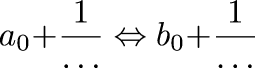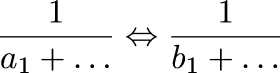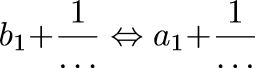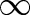Next Continued Fraction Arithmetic 44

# Comparing continued fractions is easy

• Like decimals, continued fractions are easy to compare!

• Recall:

• Is 100/89 more or less than 244/217?

• 100/89 = [1; 8, 11]

• 244/217 = [1; 8, 27]• Since the 1/... parts are <1, we can resolve this immediately if a0b0

• Otherwise, the answer is the same as:• Which is just:• Summary: compare place by place until you find a mismatch

• If the first mismatch is in an even position, invert the sense of the result

```        0    = 
1/8  = [0; 8]
2/8  = [0; 4]
3/8  = [0; 2, 1, 2]
4/8  = [0; 2]
5/8  = [0; 1, 1, 1, 2]
6/8  = [0; 1, 3]
7/8  = [0; 1, 7]
1    = 
```
• If one fraction ends prematurely, that's just like encounteringNext Back